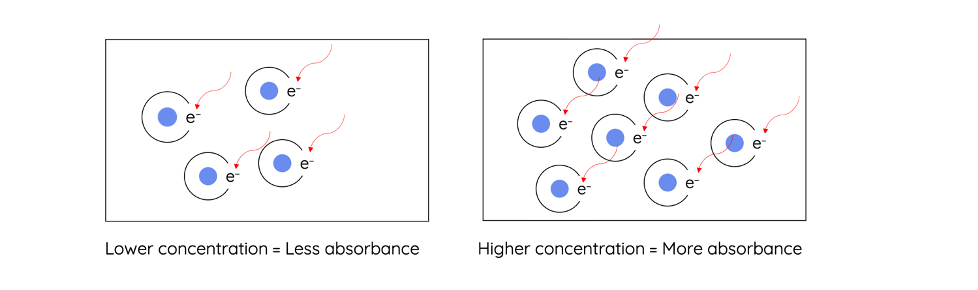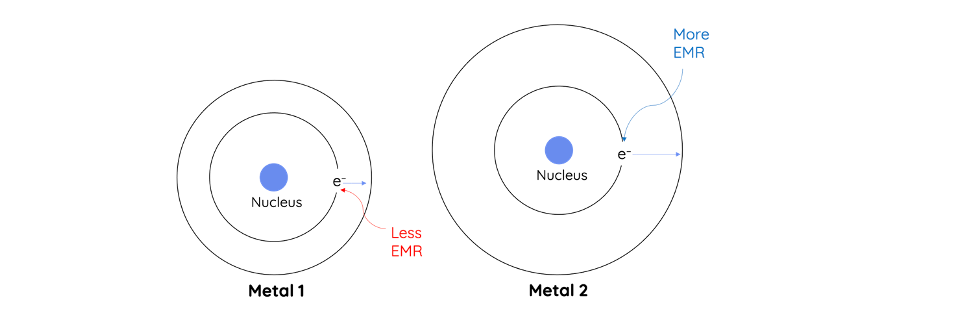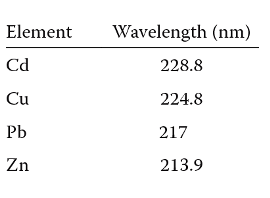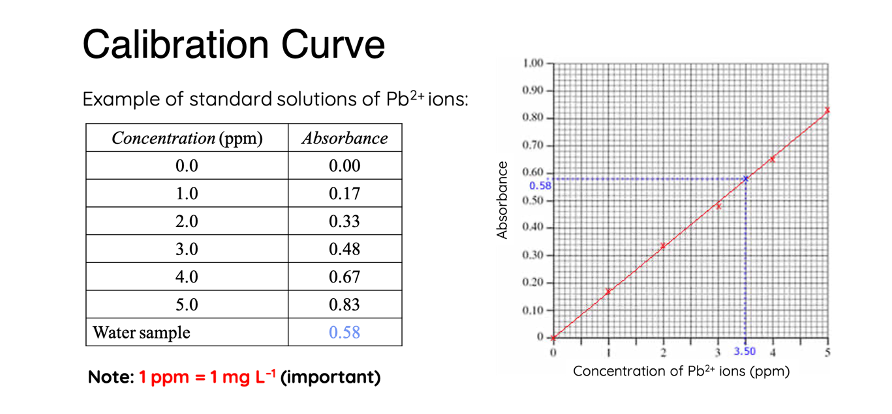# What is Atomic Absorption Spectroscopy?

This topic is part of the HSC Chemistry course under Module 8 Section 1: Analysis of Inorganic Substances.

### What is Atomic Absorption Spectroscopy (AAS)?

This video introduces atomic absorption spectroscopy (AAS). AAS is a quantitative technique used to measure the concentration of metal ions.

## What is Atomic Absorption Spectroscopy?

• Atomic absorption spectroscopy is a quantitative technique that determines the concentration of free metal ions by measuring its absorbance of electromagnetic radiation e.g. light.

##• Absorbance can be used to determined:
• Type of metal ion present in a sample
• Concentration of a particular metal ion based on the degree of absorbance. Concentration is directly proportional to absorbance (Beer’s Law)

$$A = \varepsilon cl = log_{10}(\frac{I_0}{I})$$

• AAS is a very sensitive method that can detect and measure low concentrations of metal ions. Concentrations of metal ions can be as low as a few parts per million (ppm).• AAS is a very specific technique as it can measure the concentration of a particular metal species when it is present in a solution that also contains other metals. This is because a metal atom absorbs a characteristic wavelength of radiation that matches the energy difference between orbits.

## Components in AAS

• A compound containing the metallic analyte must be passed through an atomiser (nebuliser) to be converted into free gaseous metallic ions. There are numerous ways atomisation can be achieved:
• Flame atomisers
• Electrothermal atomisersMetal ions absorb different wavelengths of radiation due to the differences in energy between their orbits. For example, an electron in a lead atom absorbs a characteristic wavelength of 217 nm. Hollow-cathode lamp releases radiations characteristic of the metallic analyte. The emitted wavelength corresponds to the analyte’s absorption spectrum.• The monochromator isolates the wavelength of radiation emitted from the hollow-cathode lamp. It ensures only the desired wavelength is detected and analysed.• Similar to other spectroscopy techniques, a standard (calibration) curve is required. This is obtained by measuring the absorbance of several dilutions of metal ions sample (with known concentration).
• AAS is commonly used to detect the presence and measure the concentration of metal ions in:
• the environment e.g. waterways
• food industry e.g. zinc supplements
• geology e.g. mineral composition